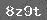# 混合运算习题精选（二）

1. 单选题

8×(6+3)的计算过程, 正确的是： [ ]

A. 8×(6+3)　　B. 8×(6+3)

=48+3　　　    　 =9×8

=51　　　　　　=72

C. 8×(6+3)　　D. 8×(6+3)

=8×9　　　　 =8×9

=72　　　　　 =72

2. 在○里填上“>”“<”或“=”．

2×(14-8)○12　　　(7-5)×6○23

3. 填空4. 先计算，再比较下面两题的运算顺序和计算结果．

12+15÷3　　　　(12+15)÷3

5. 王老师买了8根跳绳，每根7元，用去多少元? 王老师带了80元，还剩多少元?

### 相关推荐

 留言与评论（共有 0 条评论）

 验证码：